Wasinarom, K., and Charoensuk, J. (2019). "Experiment and numerical modeling of stratified downdraft gasification using rice husk and wood pellet," BioRes. 14(3), 5235-5253.

#### Abstract

Stratified downdraft gasification using rice husks and wood pellets was carried out under different air mass flow rates using both experimental and numerical methods. The flame propagation rate was calculated from the temperature profile at different time steps and was used as the prerequisite to calculate the equivalent ratio in modeling the combustion zone. Chemical equilibrium modeling was employed to predict the temperature and composition of the sample in the combustion zone. Finite kinetic modeling was used to simulate the reduction zone. The initial temperature and composition of the reduction zone simulation were obtained from the chemical equilibrium results taken from the combustion zone. The flame propagation speed of the rice husk was found to be around five times greater than wood pellet at the same air flow rate. The peak temperature of both fuels had similar values. For all air mass flow rates, the equilibrium modeling over-estimated the peaks in comparison with the experimental tests. The kinetic model was sensitive to the input temperature at the zone inlet. The predicted temperature in the reduction zone demonstrated high kinetic activity at the top of the zone due to a high gas temperature. The predicted temperature was in agreement with the experimental test results.

Experiment and Numerical Modeling of Stratified Downdraft Gasification Using Rice Husk and Wood Pellet

Kittipass Wasinarom and Jarruwat Charoensuk *

Stratified downdraft gasification using rice husks and wood pellets was carried out under different air mass flow rates using both experimental and numerical methods. The flame propagation rate was calculated from the temperature profile at different time steps and was used as the prerequisite to calculate the equivalent ratio in modeling the combustion zone. Chemical equilibrium modeling was employed to predict the temperature and composition of the sample in the combustion zone. Finite kinetic modeling was used to simulate the reduction zone. The initial temperature and composition of the reduction zone simulation were obtained from the chemical equilibrium results taken from the combustion zone. The flame propagation speed of the rice husk was found to be around five times greater than wood pellet at the same air flow rate. The peak temperature of both fuels had similar values. For all air mass flow rates, the equilibrium modeling over-estimated the peaks in comparison with the experimental tests. The kinetic model was sensitive to the input temperature at the zone inlet. The predicted temperature in the reduction zone demonstrated high kinetic activity at the top of the zone due to a high gas temperature. The predicted temperature was in agreement with the experimental test results.

Keywords: Stratified downdraft; Chemical equilibrium; Reduction zone; Gasification; Modeling

Contact information: Department of Mechanical Engineering, Faculty of Engineering, King Mongkut’s Institute of Technology Ladkrabang, Bangkok 10520, Thailand;

*Corresponding author: jarruwat.ch@kmitl.ac.th

INTRODUCTION

Thailand is an agricultural country with a distribution of residual biomass over areas of cultivation. This biomass can be used as an energy resource given that there are technologies available for economical and environmentally friendly utilization. Gasification is the thermo-chemical process of converting a biomass fuel to a combustible gas mixture. The process comprises varying degrees of oxidation, pyrolysis, and char heterogeneous reactions. It is governed by heat and the mass transfer of the specific gasification type. The reactors are categorized into fixed bed, moving bed, fluidized bed, and entrained flow. The fixed bed has a simple design, and are more appropriate for small-scale applications (Guangul et al. 2012). The combustion front propagation within packed fuel bed is the key indicator for successful operation. There are various types of the fixed bed gasification reactors, which are updraft, downdraft, and cross draft. In each case, the name indicates the flow direction of the gasification agent in relation to the fuel bed.

An updraft reactor produces a higher amount of tar in comparison to downdraft reactor because the pyrolysis gas passing through the low temperature region does not allow tar cracking to occur. Therefore, it is widely used with low-volatility fuels. More complicated systems are found in larger-scale updraft gasification. Cerone et al. (2017) conducted the oxy-steam gasification of hydrolytic residues from biorefinery. They reported H2/CO ratios as high as 2.08. Moreover, they found that using steam as the co-gasification agent would benefit in reducing temperatures above the fuel grate, therefore alleviating slag clogging from ash melting.

Downdraft gasification provides lower tar content due to higher temperatures along the pyrolysis gas flow path, which enable the tar cracking process and also allow the water-gas shift reaction, which leads to increasing combustible syn-gas. Downdraft models feature a combustion zone for volatile combustion, and the reduction zone exists underneath it.

According to Reed and Das (1998) there are two different configurations of downdraft gasification, which are the Imbert downdraft and stratified downdraft versions. There has been research on flame propagation under downdraft conditions. Dasappa and Pual (2001) found that the critical air mass flux that provides the peak propagation rate is 0.1 kg/m2 for the char bed and that the smoldering velocity will decrease with the increase of air flow rates beyond the peak value. Further increasing the air mass flux will result in flame quenching due to convection loss. Mahapatra et al. (2014) concluded that front velocity was directly correlated with bulk density of the fuel bed. However, it is difficult to specify a single parameter that influences the propagation velocity.

Combustion modeling of solid fuels, such as a biomass particle, is comprised of four sub-processes that simultaneously occur in varying degrees of kinetic rates, which are drying, devolatilization, volatiles homogeneous combustion, and char glowing or heterogeneous combustion. By employing the Arrhenius law, the estimated kinetic rates of each process can be calculated (Blasi 2000). Determining the local temperature and concentrations of related species is a pre-requisite prior to the rate calculation. In the gasification reactor, temperature and concentration distribution are governed by heat and mass transfer. The calculation procedure incorporates many inter-links of fluid dynamics, heat transfer, species transport, and the kinetic rates into the model. The solution is obtained by the iterative method.

By treating the domain as a continuum porous matrix with defined void fraction of the packed fuel bed, the heat transport equation of the packed solid fuel and the gas flow in pores space can be modelled. The calorific equations of state is comprised of Arrhenius rate of reactions, species generation from the combination of reactions, pyrolysis, and heat generation from oxidation. The domain was discretized into small finite volumes. All transport equations were repeatedly solved and updated along with the calorific equation of state of each control volume until the converged solution was obtained. This is known as conventional computational fluid dynamics (CFD) modeling. Many researchers have used CFD modeling to predict the process that occurs in the gasification reactor (Wang et al. 2014; Monteiro et al. 2017; Murugan and Sekhar 2017; Yan et al. 2018). CFD enables investigators to explore the most elaborate details of the process in different locations in the reactor.

However, the development of a robust and reliable mathematical model is required. It must be validated and calibrated with a wide range of operation. Moreover, the gasification process is a complex system with many non-linear source terms. Therefore, the CFD procedure consumes larger computational resources in comparison with other prediction methods.

The thermodynamics equilibrium model is another prediction method. The concept stems from the second law of thermodynamics. It has been widely used to evaluate the performance of gasification systems and usually developed with an assumption that the process is a lumped system. Therefore, it is widely adopted to simulate sub-zone volatile combustion in the reactor. Many researchers have used equilibrium modeling in gasification analysis (Zainal et al. 2001; Jarungthammachote and Dutta 2007; Huang and Ramaswamy 2011; Shabbar et al. 2013; Liu et al. 2016; Atnaw et al. 2017; Ferreira et al. 2019).

The reduction kinetic model was extensively used in downdraft gasification, particularly to simulate char reduction zone. The pyrolysis and combustion were assumed to be completed within the combustion zone. Because there was no oxygen in this zone, the packed fuel particle was completely transformed into char. The reduction kinetic model has been used in various research for reduction zone prediction. The predicted temperature profile and the exit gas composition were in good agreement with the experimental data (Giltrap et al. 2003). The improvement was made by using variable char reactivity factor (exponentially increasing) along the length of reduction zone (Babu and Sheth 2006). Sharma (2001) compared equilibrium modeling and finite kinetic modeling in the char reduction zone and pointed out the similar trend of the calorific values, conversion efficiency and exhaust gas temperature. Kinetic modeling was applied to finite sub-layers in the reduction zone. A number of researchers have used multi-zone modeling in their works (Gao et al. 2008; Masmoudi et al. 2014; Salem and Paul 2018). More elaborate study on the kinetic of steam gasification of various biomass chars has been performed. It was found that the char conversion rate depended on inorganic elements of the feedstock. Potassium acts as the catalyst which increases the reactivity during char conversion. On the contrary, silicon and phosphorous behave as inhibitors, which reduce the conversion rate. Dupont et al. (2016) revealed that the reactivity among different biomass chars were correlated well with the inorganic elements of potassium/(silicon+ phosphorus) ratio. They also compared the experimental result and the model prediction of biomass char conversion by using inorganic element ratio as an indicator. Prestipino et al. (2018) constructed the model prediction for different biomass char. He postulated that the Avrami-Erofeev model could capture sigmoidal behavior of some types of biomass char conversion.

There has been recent research study on the pellet used in energy plants. For instance, Bartocci et al. (2018b) made the series of experimental studies on the pyrolysis of commercial biomass pellet, followed by steam gasification of obtained charcoal pellet. The investigation focused on heat and mass balance of the integrated plant of hydrogen-rich gas production. They demonstrated that all the heat required by all different reactors can be supplied by heat released from volatile oxidation. Bartocci et al. (2018a) studied the pyrolysis of the pellet made of raw glycerol and biomass with different mixing ratio. They concluded that glycerol pellet was a good fuel to be used in Combine Heat and Power (CHP) plants.

In this paper, the interaction between flame propagation, combustion zone temperature, and reduction zone was highlighted. The flame propagation was analyzed under stratified downdraft gasification in different operating conditions with two different feedstocks, rice husk and wood pellet. Equilibrium modeling was employed to predict temperature and gas composition in the combustion zone, and kinetic reduction modeling was used to simulate the reduction zone. The analysis focused on heat and mass transfer at the propagation front and temperature distribution in the reduction zone. The predicted temperature was considered relative to the experimental data.

EXPERIMENTAL

Methods

Fixed bed reactor

The experimental test rigs are shown in Fig. 1. The reactor was made of cylindrical stainless steel tubes. It had an inner diameter of 21 cm and a height of 100 cm and was covered with 5 cm of ceramic fiber to prevent major heat loss. An air distributor manifold was located on top of the reactor. It was piped with the air compressor tank via an air flow meter. Ten type-K thermocouples (T1 through T10) were placed along the axial location. There were 10 cm gaps between the thermocouples. A thermocouple (T0) was placed at the syngas exit port. The temperature was recorded by a YOKOGAWA FX100 data recording system (Yokogawa Electric Corporation, Tokyo, Japan). A metering rod used for monitoring bed height was placed on the top of the reactor.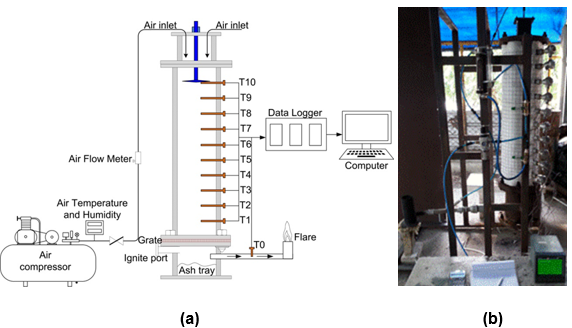Fig. 1. Schematic diagram for downdraft gasification (a) and the reactor used for downdraft gasification (b)

Experimental procedure

The feedstocks were poured into the reactor from the top. In the case of rise husk, 3 kg was poured, while 20 kg was used in the case of wood pellet. The top surface of the packed fuel was meant to reach a height of 100 cm if it was properly packed into the reactor. Air was fed into the reactor, and the burner was used for firing into the ignite port. T1 was monitored until it reached a temperature of 450 K. The volatile combustion took place and propagated at this temperature. After that, the burner was pulled out and the ignite port was closed. The air input was adjusted to satisfy specific cases of the experiment. A data logger was used to collect temperature information at intervals of 5 min. The peak temperature moved in the upward direction following the propagation front during the test period, as shown in Fig. 3. Finally, the propagation front reached the top surface of the packed fuel bed. This was indicated by movement of the peak temperature in the downward direction to the grate below. This behavior is further explained in results and discussion section. At this moment, the air supply and data logger were stopped. The total bed movement was measured by the bed height indicator rod. The residual was collected and weighted to perform the mass loss calculation. An experimental test case was completed for a specified condition. In this work, 6 cases of the experimental were performed. The details of all conditions are provided in Table 1.

Table 1. Experimental Details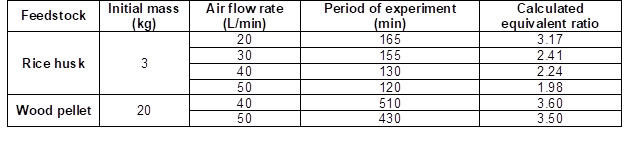Materials

Wood pellet (Jumneansawas 2017) and rice husk (Madhiyanon et al. 2010) were used as fuel in this work. Their properties and heating values are shown in Table 2.

Table 2. Proximate and Ultimate Analyses of Wood Pellet and Rice Husk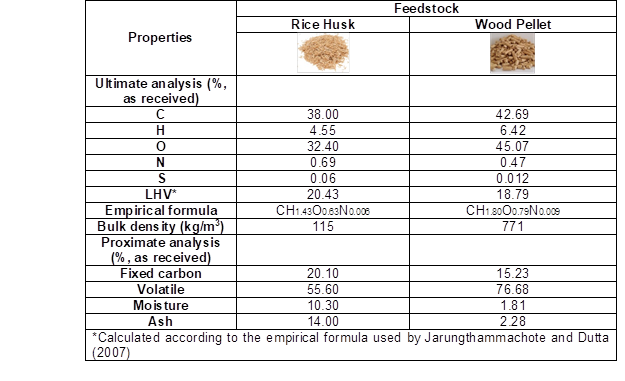The flame front propagation rate (FPR) was calculated by tracing the location of the peak temperature along the recorded period of time between two adjacent thermocouples (Mahapatra et al. 2014), as indicated below.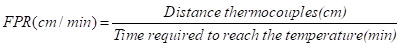(1)

Bed movement was calculated using Eq. 2.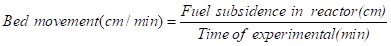(2)

Modeling

Equilibrium modeling

The global species balance for gasification of dry feedstock is expressed by Eq. 3,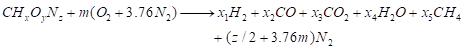(3)

where x, y, and z are the atomic numbers of hydrogen, oxygen, and nitrogen in the feedstock, respectively, and x1 to x5 represent the moles of the gasification products per 1 mole of feedstock. The variable m represents (4.76 m) the moles of air that were introduced in gasification per 1 mole of feedstock. The atomic balance of carbon, hydrogen, and oxygen atoms could be written in Eqs. 4, 5, and 6, respectively, as shown below.

Carbon balance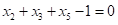(4)

Hydrogen balance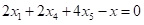(5)

Oxygen balance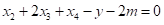(6)

There are three major kinetics that contribute to gasification process which are the Boudouard reaction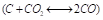2.), CO water shift reaction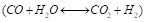, and methanation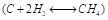. The equilibrium constants of each kinetic rate are expressed by Eqs. 7, 8, and 9,

Equilibrium constant for CO water shift reaction: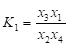(7)

Equilibrium constant for methanation combustion,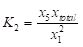(8)

where xtotal is the total molar of the gaseous product from Eq. 3, which can be calculated by Eq. 9.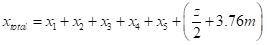(9)

The equilibrium constants of any kinetic rates can be calculated from Eq. 10. The parameter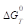is the change in Gibbs function of formation obtained from all species involved in the reaction, which are functions of temperature. The calculation of the Gibbs of formation are referred to by Jarungthammachote and Dutta (2007).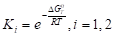(10)

The energy equation could be written in the form of Eq. 11. The temperature of gas compositions can be calculated by energy conservation principle.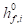is the enthalpy of formation of the reactant; (kJ/kmol).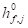is the enthalpy of formation of the products (kJ/kmol).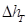is the sensible enthalpy of the products (kJ/kmol).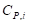is the specific heat of the ith species in the product, which is a function of temperature (kJ/kmol K). It was calculated in accordance with temperature by using the correlation referred to by Jarungthammachote and Dutta (2007).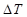is the change in temperature with respect to the reference temperature of 298 K.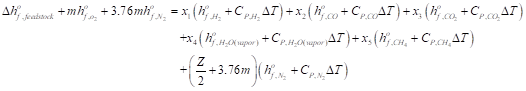(11)

Reduction kinetic modeling

The reduction kinetic rate model was described in previous studies (Sharma 2001; Giltrap et al. 2003). The heat of reaction and the reaction rate of all considering reactions can be written as follows,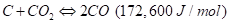(12)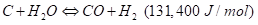(13)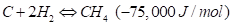(14)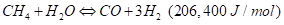(15)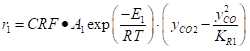(16)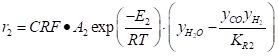(17)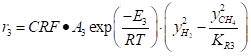(18)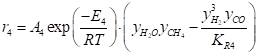(19)

where ri is the rate of reaction for the ith reaction; (kmol/m3s), Ai is the frequency factor for the ith reaction; (kmol/m3s), Ei is the activation energy of the ith reaction(kj/kmol). R is the universal gas constant; (kj/kmol), T is the temperature, yx is the mole fraction of x species, ki is the equilibrium constant for the ith reaction, and CRF is the char reactivity factor.

Model solution procedure

The flow chart of the numerical procedure is illustrated in Fig. 2. The chemical equilibrium modeling was employed to predict the temperature and the composition of the combustion zone. Then the obtained temperature and composition was used as the initial value in reduction kinetic modeling in the reduction zone. In obtaining the solution for the chemical equilibrium modeling, there was a need to couple between an energy equation and the system of chemical equilibrium equations. This could be achieved through an iterative technique until the relative error of the predicted temperature was at an acceptable tolerance. For the solution of reduction kinetic modeling, the finite layer height was defined in the reduction zone and the residence time in each layer was estimated. The temperature and the composition were then used as the input for the adjacent layer below. More detail of the zone definition from the experimental information will be discussed in the next section.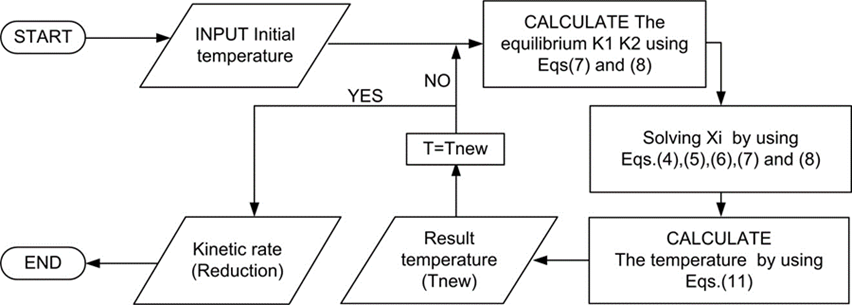Fig. 2. Calculation procedure

RESULTS AND DISCUSSION

Flame Propagation and Kinetic Mechanism in Stratified Downdraft Gasification

The flame propagation and characteristics of each regime of the stratified downdraft gasification in different conditions were similar. It comprised of a combustion zone at the flame front following by a reduction zone, as depicted in Fig. 3a. The steep temperature gradient where the temperature rose to its peak was observed in the combustion zone. This was due to major heat release from volatile combustion within the pores of the packed fuel bed. Oxygen was completely consumed within this zone. The fresh fuel was devolatilized, thus leaving char behind as the flame propagation moved counter to the supplied oxidizer stream as shown in Fig. 3b.

The reduction zone was located below the combustion zone. In this zone, the kinetic mechanism took place in the absence of the oxidizer. Flue gas from the combustion zone then reacted with solid char at a high temperature to produce carbon monoxide and hydrogen. While carbon dioxide and steam were consumed in the reduction process, the process was endothermic overall, so a decrease in temperature along the direction of the flue gas was observed in this zone.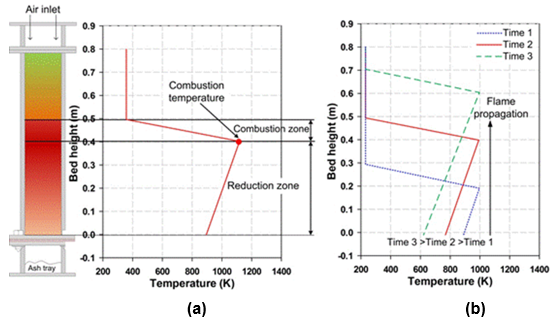Fig. 3. Zone and temperature at specific times (a) and zone and temperature movement at different time periods (b)

Flame Propagation in Stratified Downdraft Gasification

In stratified downdraft gasification conditions, flame propagation moved counter to the supply oxidizer stream, as calculated by Eq. 1. The speed of the propagation depended on various factors, for example, fuel particle type, fuel size, moisture content in fuel, and air mass flow rate. The flame propagation mechanism was controlled by diffusion heat and mass transports in the direction counter to the supplied oxidizer stream. This was observed at the reaction front where a steep temperature gradient was presented. There were two distinctive regimes revealed in the propagation characteristic regardless the type of feedstock.

In regime I, at a low range of air flow rate, the propagation speed increased with increasing air flow rates. As the air flow rate increased, at a certain point, the peak propagation speed was reached. In regime II, at a higher range of air flow rate, propagation speed decreased with increasing air flow rate (Sadhan et al. 2014). In this scenario, increasing the air flow rate more than that resulted in the peak propagation speed. The propagation speed has a direct variation with the peak temperature under different air flow rates and the same feedstock conditions (Onthong et al. 2016).